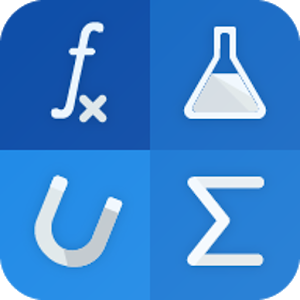Español

Todos los días ofrecemos apliaciones y juegos licenciados GRATIS para Android de otra manera tendrás que comprarlo.1.49
EXPIRÓ

# Android Giveaway of the Day - Formula Solver

Simple application for physical and chemical calculations.
1.49 EXPIRÓ
Votación de Usuario: 0 (0%) 0 (0%)

Esta oferta de regalo ha expirado. Formula Solver ya está disponible.

Simple application for physical and chemical calculations. This app help you in physics, math and chemistry, if you need to know, what formula you must use in calculation, help you to control your calculation and calculating in formula.

Categories of physics:
* Electricity and magnetism
* Hydromechanics
* Mechanics of vibration, waves and acoustics
* Mechanics
* Molecular physics and ternodynamics
* Optics
* Quantum physics
* Special theory of relativity

Mathematical formulas

* For most calculations, it is possible to show the calculation procedure
* Search in categories
* Unit converter

Preparing:
* Show calculation procedure for all formulas (Premium)
* Add a formula to the test section
* Math formulas

The icons in the application come from www.materialdesignicons.com

The application icon is from Martin Šenovský - https://www.facebook.com/SenovskyMartin/

David Šimák

Education

3.0.0

### Tamaño:

3.1M

Everyone